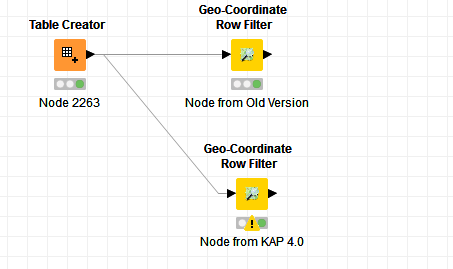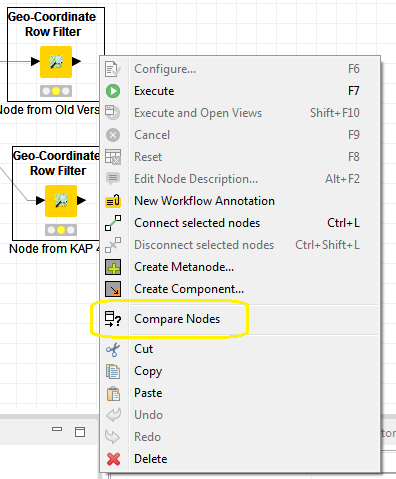# KNIME Analytics Platform 4.0 : Geo-Coordinate Row Filter issues

Hi Guys,
I am using KNIME Analytics Platform(KAP) 4.0. I have a workflow that was created in an earlier version of KNIME in which I use Geo-Coordinate Row Filter(GCRF). The workflow runs all good on KAP 4.0 but if I try to use a new GCRF node from the Node Repository, then the same setup returns empty data set. This seems to be kind of weird. Have you encountered such issues with KAP 4.0?Geo-Coordinate Row Filter Issues.knwf (9.9 KB)The two nodes are configured differently:

``````algebraic:Geo_Coordinate Row Filter (#1761) loki\$ diff ../Geo_Coordinate\ Row\ Filter\ \(#2264\)//settings.xml settings.xml
24,29c24,28
< <entry key="array-size" type="xint" value="5"/>
< <entry key="0" type="xdouble" value="-454.21875"/>
< <entry key="1" type="xdouble" value="-413.7890625"/>
< <entry key="2" type="xdouble" value="-413.96484375"/>
< <entry key="3" type="xdouble" value="-452.28515625"/>
< <entry key="4" type="xdouble" value="-454.130859375"/>
---
> <entry key="array-size" type="xint" value="4"/>
> <entry key="0" type="xdouble" value="-75.41677057743073"/>
> <entry key="1" type="xdouble" value="-75.41489839553833"/>
> <entry key="2" type="xdouble" value="-75.41489839553833"/>
> <entry key="3" type="xdouble" value="-75.41709244251251"/>
32,37c31,35
< <entry key="array-size" type="xint" value="5"/>
< <entry key="0" type="xdouble" value="47.45780853075031"/>
< <entry key="1" type="xdouble" value="47.989921667414194"/>
< <entry key="2" type="xdouble" value="30.14512718337613"/>
< <entry key="3" type="xdouble" value="30.372875188118016"/>
< <entry key="4" type="xdouble" value="30.44867367928756"/>
---
> <entry key="array-size" type="xint" value="4"/>
> <entry key="0" type="xdouble" value="40.09760279901132"/>
> <entry key="1" type="xdouble" value="40.09718424044669"/>
> <entry key="2" type="xdouble" value="40.096076278877625"/>
> <entry key="3" type="xdouble" value="40.09685185387012"/>
51c49
< <entry key="text" type="xstring" value="Node from KAP 4.0"/>
---
> <entry key="text" type="xstring" value="Node from Old Version"/>
53,55c51,53
< <entry key="x-coordinate" type="xint" value="292"/>
< <entry key="y-coordinate" type="xint" value="358"/>
< <entry key="width" type="xint" value="106"/>
---
> <entry key="x-coordinate" type="xint" value="278"/>
> <entry key="y-coordinate" type="xint" value="219"/>
> <entry key="width" type="xint" value="124"/>
algebraic:Geo_Coordinate Row Filter (#1761) loki\$
``````
2 Likes

Hi there,

You can compare nodes in KNIME to check this.Both GCRF nodes are from same version although one is from workflow created with older KNIME versionBr,
Ivan

2 Likes

@quaeler : The only difference that was between the 2 nodes was that I included a much larger area in the node created within KAP 4.0 . Is that what you are referring to. Appreciate your time and efforts.

I have almost no knowledge of this node itself, so i’m only pointing out that there is a difference in configuration and there is no other difference (and so therefore something in this configuration difference is producing an empty table versus non-empty table.)

1 Like

This topic was automatically closed 182 days after the last reply. New replies are no longer allowed.